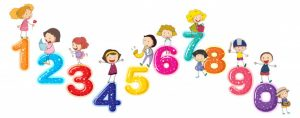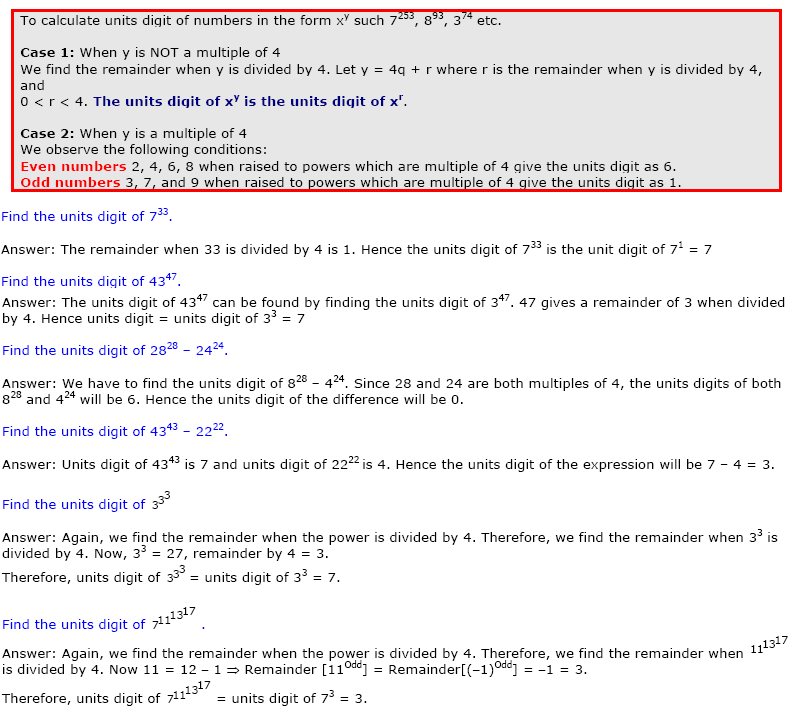# Last Digit / Units DigitTo find the units digit of xy we only consider the units digits of the number x.
To calculate units digit of 237234 we only consider the units digit of 237. Hence, we find the units digit of 7234.
To find the units digit of a × b, we only consider the units digits of the numbers a and b.
To calculate units digit of 233 ×  254, we only consider the units digit of 233 and 254 i.e. 3  and 4, respectively. Hence, the units digit of 233 ×  254 = units digit of 3 × 4 i.e 2.### Problems for practice

1. Find the unit digit of [ 1020000 / ( 10100 +3) ] where [ x ] is greatest Integer less than or equal to x.
2. Find the unit digit of the product of first 120 primes .
3. What is the unit digit of 1! + 2! + 3! + 4! +….. + 2018! ?
4.  What is the unit digit of (1! + 2! + 3! + 4! +….. + 2018!)2?
5. What is the unit digit of 231001 ?

#### Some more ‘Gyan’ on numbers :Nifty Formulae for Natural NumbersDivisibility Rule of a Number (Divisibility Test)Remainders theorem Divisors of a Number ( Number of Divisors of a number)

Do you know that we have a forum where you can get all your doubts cleared?
Forums